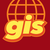# Creating / updating feature layer in ArcGIS Online from geopandas GeoDataFrame?

980
4
02-13-2019 11:21 PMNew Contributor III

Hi

Is it at all possible to create a feature layer in ArcGIS Online from a GeoDataFrame? If you cannot create a feature layer directly from a GeoDataFrame, is it possible to populate an existing feature layer from a GeoDataFrame (provided the fields match of course).

I tried all sorts of methods to create a feature layer directly using GeoJSON as the intermediary  - no luck. So then I tried to insert values (attributes and geometry) from a GeoDataFrame into an existing feature layer in AGOL, using GeoDataFrame.to_dict and then trying to add that dictionary to the feature layer using edit_features(adds=<my dictionary created from the GeoDataFrame>). No luck there either.

Anyone knows how this can be done?

Thanks

Hugo

Tags (5)
4 RepliesOccasional Contributor II

Yep you can using this method:New Contributor III

Here's a method I wrote for GeoDataFrame to return an arcgis spatially-enabled dataframe (SEDF):

``import warningsfrom arcgis.geometry import Geometryfrom arcgis.features import GeoAccessor, GeoSeriesAccessorfrom pandas import DataFramefrom geopandas import GeoDataFramedef to_SpatialDataFrame(self, spatial_reference=None):    """Returns an arcgis spatially-enabled data frame.    Arguments:    spatial_reference  Either None or a spatial reference integer code.                       If None, the spatial reference will be extracted                       from the GeoDataFrame if it is defined using an                       EPSG code.    """    if not spatial_reference:        crs = self.crs        epsg_code = crs.to_epsg()        if epsg_code:            spatial_reference = {'wkid': epsg_code}        else:            spatial_reference = {'wkid': 4326}            warnings.warn('Unable to extract a spatial reference, assuming latitude/longitude (EPSG 4326).')    else:        spatial_reference = {'wkid': spatial_reference}    sdf = DataFrame(data=self.drop(self.geometry.name, axis=1))    sdf['SHAPE'] = [Geometry.from_shapely(g, spatial_reference) for g in self.geometry.tolist()]    sdf.spatial.set_geometry('SHAPE')    return sdfGeoDataFrame.to_SpatialDataFrame = to_SpatialDataFrame‍‍‍‍‍‍‍‍‍‍‍‍‍‍‍‍‍‍‍‍‍‍‍‍‍‍‍‍‍‍‍‍‍‍‍‍‍‍‍‍‍‍‍‍‍‍‍‍‍‍‍‍‍‍‍‍‍‍‍‍‍‍‍‍‍‍‍‍‍‍‍‍‍‍‍‍‍‍‍‍‍‍‍‍‍‍‍‍‍‍‍‍‍‍‍‍‍‍‍‍``

The resulting SEDF can then be published or used to update an existing feature layer.New Contributor III

That's very interesting, thanks!by
New Contributor II

There is a method using the arcgis api:

``GeoAccessor.from_geodataframe(geo_df, inplace=False, column_name='SHAPE')``